It's time to celebrate Pi Day! Every year on March 14th (written 3/14 in the US), math-loving folks celebrate "all things pi-related" because 3.14 is the three-decimal approximation to the mathematical constant, π. Although children learn that pi is approximately 3.14159..., the actual definition of π is the ratio of a circle's circumference to its diameter. Equivalently, it is distance around half of the unit circle. (The unit circle has a unit radius, so its diameter is 2.) The value for pi, therefore, depends on the definition of a circle.

But we all know what a circle looks like, don't we? How can there be more than one circle?

### Generalizing the circle

A circle is defined as the locus of points in the plane that are a given distance from a given point. This definition depends on the definition of a "distance," and it turns out that there are infinitely many ways to measure the distance between two points in the plane. The Euclidean distance between two points is the most familiar distances, but there are other definitions. For two points a = (x1, y1) and b = (x2, y2), you can define the "Lp distance" between a and b by the formula
Dp = ( |x1 – x2|p + |y1 – y2|p )1/p
This definition defines a distance metric for every value of p ≥ 1. If you set p=2 in the formula, you get the usual L2 (Euclidean) distance. If you set p=1, you get the L1 metric, which is known as the "taxicab" or "city block" distance.

You might think that the Euclidean distance is the only relevant distance, but it turns out that some of these other distances have practical applications in statistics, machine learning, linear algebra, and many fields of applied mathematics. For example, the 2-norm (L2) distance is used in least-squares regression whereas the 1-norm (L1) distance is used in robust regression and quantile regression. A combination of the two distances is used for ridge regression, LASSO regression, and "elastic net" regression.

Here's the connection to pi: If you can define infinitely many distance formulas, then there are infinitely many unit circles, one for each value of p ≥ 1. And if there are infinitely many circles, there might be infinitely many values of pi. (Spoiler alert: There are!)

### Would the real circle please stand up?

You can easily solve for y as a function of x and draw the unit circle for a representative set of values for p. The following graph was generated by the SAS step and PROC SGPLOT. You can download the SAS program that generates the graphs in this article.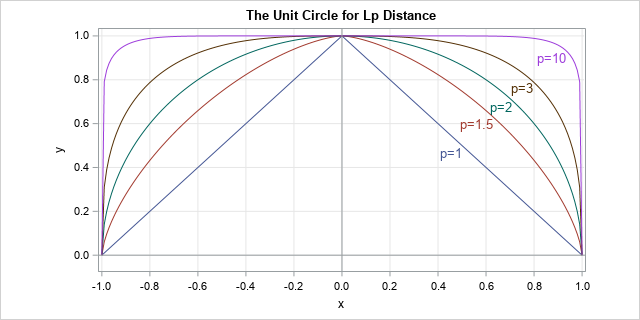The L1 unit circle is a diamond (the top half is shown), the L2 unit circle is the familiar round shape, and as p gets large the unit circle for the Lp distance approaches the boundary of the square defined by the four points (±1, ±1). For more information about Lp circles and metrics, see the Wikipedia article "Lp Space: The p-norm in finite dimensions."

Here comes the surprise: Just as each Lp metric has its own unit circle, each metric has its own numerical value for pi, which is the length of the unit semicircle as measured by that metric.

### π(p): The length of the unit semicircle for the Lp distance metric

So far, we've only used geometry, but it's time to use a little calculus. This presentation is based on Keller and Vakil (2009, p. 931-935), who give more details about the formulas in this section.

For a curve that is represented as a graph (y as a function of x), you can obtain the length of the curve by integrating the arclength. In Calculus 2, the arclength formula is derived for Euclidean distance, but it is straightforward to give the formula for the Lp distance:
s(p) = ∫ (1 + |dy/dx|p)1/p dx

To obtain a value for pi in the Lp metric, you can integrate the arclength for the upper half of the Lp unit circle. Equivalently, by symmetry, you can integrate one-eighth of the unit circle and multiply by 4. A convenient choice for the limits of integration is [0, 2-1/p] because 2-1/p is the x value where the 45-degree line intersects the unit circle for the Lp metric.

Substituting for the derivative gives the following formula (Keller and Vakil, 2009, p. 932):
π(p) = 4 ∫ (1 + u(x))1/p dx, where u(x) = |x-p - 1|1-p and the interval of integration is [0, 2-1/p].

### A pi for each Lp metric

For each value of p, you get a different value for pi. You can use your favorite numerical integration routine to approximate π(p) by integrating the formula for various values of p ≥ 1. I used SAS/IML, which supports the QUAD function for numerical integration. The arclength computation for a variety of values for p is summarized by the following graph. The graph shows the computation of π(p), which is the length of the semicircle in the Lp metric, versus values of p for p in [1, 11].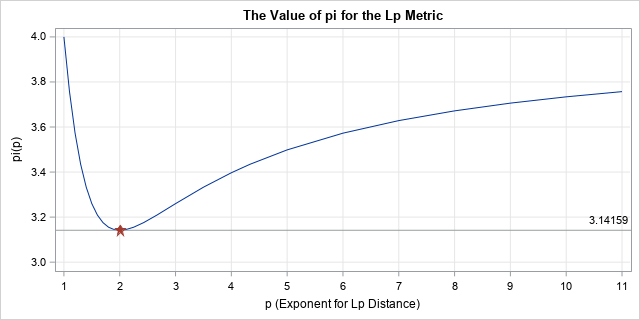The graph shows that the L1 value for pi is 4. The value decreases rapidly as p approaches 2 and reaches a minimum value when p=2 and the value of pi is 3.14159.... For p > 2, the graph of π(p) increases slowly. You can show that π(p) asymptotically approaches the value 4 as p approaches infinity.

On Pi Day, some places have contests to see who can recite the most digits of pi. I encourage you to enter the contest and say "Pi, in the L1 metric, is FOUR point zero, zero, zero, zero, ...." If they refuse to give you the prize, tell them to read this article! 😉

### Reflections on pi

One the one hand, this article shows that there is nothing special about the value 3.14159.... For an Lp metric, the ratio of the circumference of a circle to its diameter can be any value between π and 4. On the other hand, the graph shows that π is the unique minimizer of the graph. Among an infinitude of circles and metrics, the well-known Euclidean distance is the only Lp metric for which pi is 3.14159....

If you ask me, our value of π is special, without a doubt!

### References

The post The value of pi depends on how you measure distance appeared first on The DO Loop.Welcome to my annual Pi Day post. Every year on March 14th (written 3/14 in the US), geeky mathematicians and their friends celebrate "all things pi-related" because 3.14 is the three-decimal approximation to pi.

Pi is a mathematical constant that never changes. Pi is the same value today as it was in ancient Babylon and Greece. The timeless constancy of pi is a comforting presence in a world of rapid change.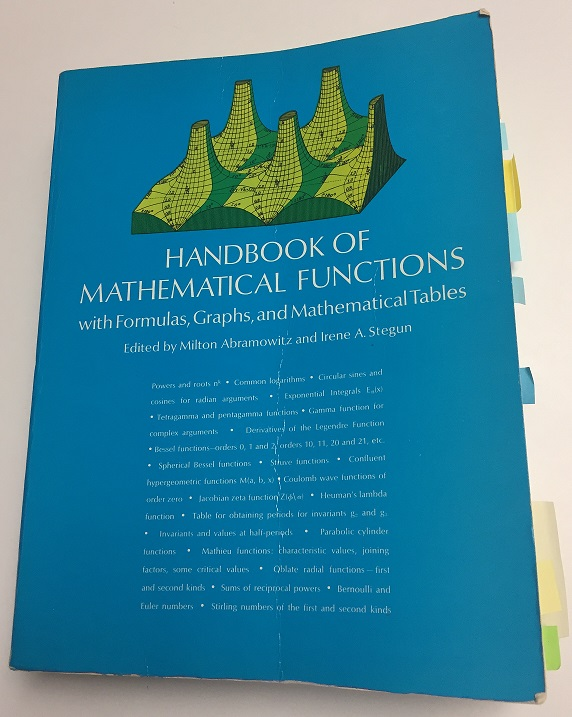But even though the value of pi does not change, our knowledge about pi does change and grow. I was reminded of this recently when I opened my worn copy of the Handbook of Mathematical Functions (more commonly known as "Abramowitz and Stegun," the names of its editors). When the 1,046-page Handbook was published in 1964, it was the premier reference volume for applied mathematicians and mathematical scientists. Interestingly, pi is not even listed in the index! It does appear on p. 3 under "Mathematical Constants," which gives a 25-digit approximation of many mathematical constants such as pi, e, and sqrt(2).

### How to define pi?

Fast forward to the age of the internet. In 2010, the Handbook was transformed into an expanded online, searchable, interactive web site. The new Handbook is called The NIST Digital Library of Mathematical Functions. This is very exciting because the Handbook is now available (for free!) to everyone!

If you search for pi in the online Digital Library, you find that the editors chose to define pi as the value of the integral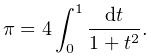This seems to be a strange way to define pi. Pi is the ratio of the circumference and diameter of a circle, and upon first glance that formula doesn't seem related to a circle. A more geometric choice would be an integrand such as sqrt(1 + t2), which connects pi to the area under the unit circle.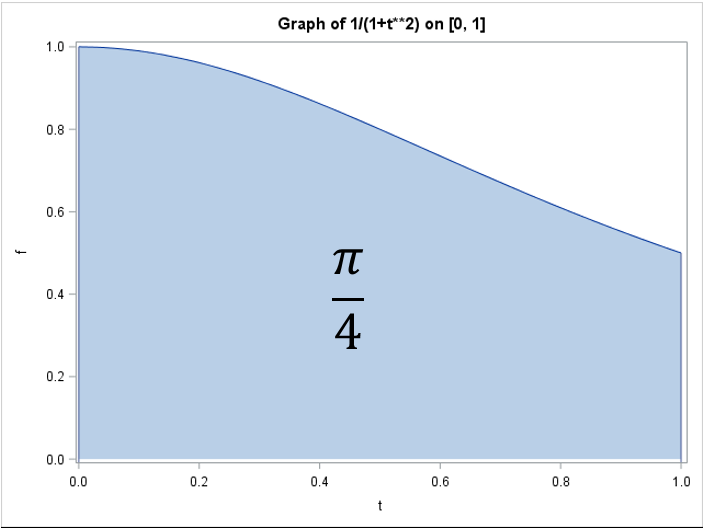Of course, the integral in the Digital Library is equal to pi, but it is not obvious. You might recall from calculus that the antiderivative of 1/(1+t2) is arctan(t). Therefore the expression is just a complicated way to write 4 arctan(1). Ah! This makes more sense because arctan(1) is equal to π/4. In fact, before SAS introduced the CONSTANT function, SAS programmers used to define pi by using the computation pi = 4*ATAN(1). Nevertheless, I think expressing arctan(1) as an integral is unnecessarily obtuse.

### Using the Cauchy distribution to define pi

I am not enamored with the editors' choice of an integral to define pi, but if I were to use that integrand to define pi, I would use a variation that has applications in probability and statistics. Statisticians sometimes use the Cauchy distribution, which is a fat-tailed distribution that has the interesting mathematical property that the distribution has no mean (expected) value! (Mathematicians say that "the first moment does not exist.") Researchers in robust statistical methods sometimes use Cauchy-distributed errors to generate extreme outliers in simulated data.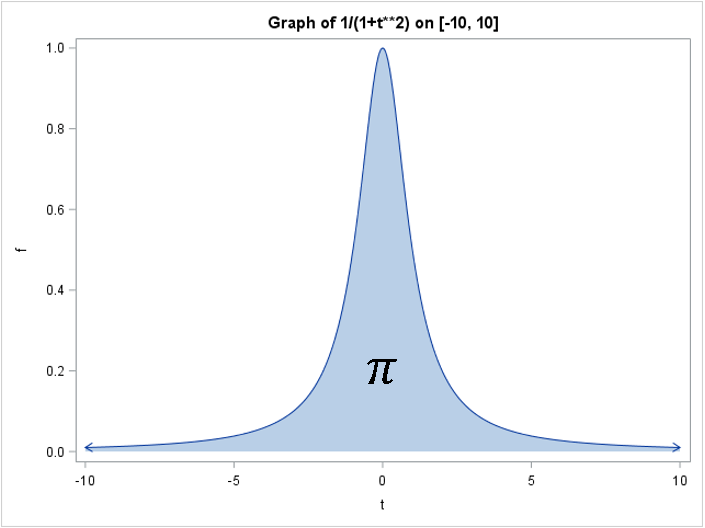The Cauchy probability density function (PDF) is 1/π 1/(1+t2), which means that the integral of the PDF on the interval [-∞, ∞] is 1. Equivalently, the integral of 1/(1+t2) on the interval [-∞, ∞] is π: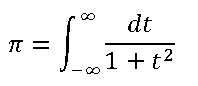This definition of pi seems more natural than the integral on [0, 1]. I could make other suggestions (such as the integral of arccos on [-1, 1]), but I think I'll stop here.

The purpose of this post is to celebrate pi, which is so ubiquitous and important that it can be defined in numerous ways. A secondary purpose is to highlight the availability of the NIST Digital Library of Mathematical Functions, which is an online successor of the venerable Handbook of Mathematical Functions. I am thrilled with the availability of this amazing resource, regardless of how they define pi!

To complete this Pi Day post, I leave you with a pi-ku. A pi-ku is like a haiku, but each line contains syllables the number of syllables in the decimal expansion of pi. A common structure for a pi-ku is 3-1-4. The following pi-ku celebrates the new Digital Library:

Handbook of
Math
Functions? Online!

The post Pi, special functions, and distributions appeared first on The DO Loop.It is time for Pi Day, 2017! Every year on March 14th (written 3/14 in the US), geeky mathematicians and their friends celebrate "all things pi-related" because 3.14 is the three-decimal approximation to pi. This year I use SAS software to show an amazing fact: you can find your birthday (or any other date) within the first 10 million digits of pi!

### Patterns within the digits in pi

Mathematicians conjecture that the decimal expansion of pi exhibits many properties of a random sequence of digits. If so, you should be able to find any sequence of digits within the decimal digits of pi.

If you want to search for a particular date, such as your birthday, you need to choose a pattern of digits that represents the date. For example, Pi Day was first celebrated on 14MAR1988. You can represent that date in several ways. This article uses the MMDDYY representation, which is 031488. You could also use a representation such as 31488, which drops the leading zero for months or days less than 10. Or use the DDMMYY convention, which is 140399.

Can you find your birthday within the digits of pi?
Click To Tweet

In 2015 I showed how to use SAS software to download the first ten million digits of pi from an internet site. The program then uses PROC PRINT to print six consecutive digits of pi beginning at the 433,422th digit:

```/* read data over the internet from a URL */ filename rawurl url "http://www.cs.princeton.edu/introcs/data/pi-10million.txt" /* proxy='http://yourproxy.company.com:80' */ ; data PiDigits; infile rawurl lrecl=10000000; input Digit 1. @@; Position = _n_; run;   /* Pi Day "birthday" 03/14/88 represented as 031488 */ proc print noobs data=PiDigits(firstobs=433422 obs=433427); var Position Digit; run;```Look at that! The six-digit pattern 031488 appears in the decimal digits of pi! This location also contains the alternative five-digit representation 31488, but you can find that five-digit sequence much earlier, at the 19,466th digit:

```/* Alternative representation: Pi Day birthday = 31488 */ proc print noobs data=PiDigits(firstobs=19466 obs=19470); var Position Digit; run;```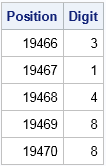How did I know where to look for these patterns? Read on to discover how to use SAS to find a particular pattern digits within the decimal expansion of pi.

### Finding patterns within the digits in pi

Last week I showed how to use SAS to search for a particular pattern within a long sequence of digits. Let's use that technique to search for the six-digit Pi Day "birthday," pattern 031488. The following call to PROC IML in SAS defines a function that implements the search algorithm. The program then reads in the first 10 million digits of pi and conducts the search for the pattern:

```proc iml; /* FindPattern: Finds a specified pattern within a long sequence of digits. Input: target : row vector of the target pattern, such as {0 3 1 4 8 8} digits : col vector of the digits in which to search Prints the number of times the pattern appears and the first location of the pattern. See https://blogs.sas.com/content/iml/2017/03/10/find-pattern-in-sequence-of-digits.html */ start FindPattern(target, digits); p = ncol(target); /* length of target sequence */ D = lag(digits, (p-1):0); /* columns shift the digits */ D = D[p:nrow(digits),]; /* delete first p rows */ X = (D=target); /* binary matrix */ /* sum across columns. Which rows contain all 1s? */ b = (X[,+] = p); /* b[i]=1 if i_th row matches target */ NumRepl = sum(b); /* how many times does target appear? */ if NumRepl=0 then FirstLoc = 0; else FirstLoc = loc(b); result = NumRepl // FirstLoc; labl = "Pattern = " + rowcat(char(target,1)); /* convert to string */ print result[L=labl F=COMMA9. rowname={"Num Repl", "First Loc"}]; finish;   /* read in 10 million digits of pi */ use PiDigits; read all var {"Digit"}; close;   target = {0 3 1 4 8 8}; /* six-digit "birthday" of Pi Day */ call FindPattern(target, Digit);   target = {3 1 4 8 8}; /* five-digit "birthday" */ call FindPattern(target, Digit);```Success! The program shows the starting location for each pattern within the digits of pi. The starting locations match the values of the FIRSTOBS= option that was used in PROC PRINT in the previous section.

### Search for your birthday within the digits of pi

You can use this program to search for your birthday, your anniversary, or any other special date. (If you prefer to use the SAS DATA step, see the comments of my previous article.) If you don't have SAS, don't despair! I got the idea for this article from a nifty web page on PBS.org that contains an applet that you can use to find your birthday among the digits of pi.

The PBS applet does not require any special software. However, I noticed that it gives slightly different answers from the SAS program I wrote. One trivial difference is that the applet starts with the "3" digit of pi, whereas the SAS program starts with the "1" in the tenths decimal place. So the two programs should give locations that differ by one place. Another difference is that the applet appears to always represent months and days that are less than 10 as a one-digit value, so that the PBS applet represents 02JAN2003 as "1203" rather than "010203." However, I have observed (but cannot explain) that the PBS applet seems to consistently report a location that is three digits more than the SAS-reported location. For example, the applet reports 02JAN2003 (1203) as occurring at the 60,875th digit, whereas the SAS program reports the location as the 60,872th digit.

### Some unique dates within the digits of pi

We know that the Pi Day "birthday" date appears, but what about other dates? I wrote a SAS program that searches for all six-digit MMDDYY representation of dates from 01JAN1900 to 21DEC1999. I verified all dates are contained in the first 10 million digits of pi except for one. The date 01DEC1954 (120154) is the only date that does not appear!

I also discovered some other interesting properties while searching for dates (in the MMDDYY format) within the first 10 million digits of pi:

1. First appearance: The first date to appear is 28JUN1962 (062862), which appears in the 71st decimal location.
2. Latest (first) appearance: The date 23NOV1960 (112360) does not appear until the 9,982,545th location.
3. Rarest: The date 01DEC1954 (120154) is the only date that does not appear. (But the five-digit representation (12154) does appear.)
4. Second rarest: There are 15 dates that only appear one time.
5. Most frequent: The date 22JUL1982 (072282) appears 25 times.
6. Distribution of appearances: Most dates appear between seven and 12 times. The following graph shows the distribution of the number of times that each date appears.If you want to discover other awesome facts, you can explore the data yourself. You can download the results (in CSV format) of the exhaustive search. If you want to see how I searched the set of all MMDDYY patterns, you can download the SAS program that I used to create the analyses in this article.

The post Find your birthday in the digits of pi appeared first on The DO Loop.Math lovers, do you know what day it is? It's Pi Day, which we celebrate every year on March 14 because the date 3-14 matches the first three digits of pi, 3.14. This year, I'm celebrating with poetry, combining my love of math with my love of language. Word Spy explains that a pi-ku is […]

Let's celebrate Pi Day with a pi-ku was published on SAS Voices.Last week, I celebrated the progress we've seen in cleaning up wayward pie charts, and today I again use Pi Day as an opportunity to give the world #OneLessPie. Since I am at Discovery Summit Europe in Amsterdam this week, I chose a European topic for my makeover. I started […]

The post #OneLessPie for Pi Day appeared first on JMP Blog.Pi Day (3/14) is next week, and once again, we are encouraging everyone in the data visualization community to use this day as motivation to help clean up ineffective pie charts within their organization or in public spaces like Wikipedia. I think there is still a mindset outside of our community that a pie […]

The post Progress on #OneLessPie appeared first on JMP Blog.With Pi Day coming up on 3/14, I wanted to make sure all you SAS programmers know how to use the pi constant in your SAS code... All you have to do is use constant("pi") in a data step, and you've got the value of pi out to a good many decimal places […]Last year, we launched the #OneLessPie initiative to use Pi Day (March 14 or 3/14) as a catalyst to improve the data visualization landscape. Many experts have criticized pie charts as a poor way to communicate information, and we listed some of the pitfalls of pie charts last year. But […]It's wonderful to see the #onelesspie effort gathering interest, especially on twitter. For my introductory post yesterday, I wanted to focus on encouraging people to improve pie charts everywhere. In this post, I want to show you how I remade the pie chart in JMP. Here's the original: The first [...]It's become too easy and common for data visualization practitioners to point to flaws in pie charts and other artless visualizations. Far better is to pair criticism with demonstrated improvements. Kaiser Fung's junkcharts blog is the pioneer in backing words with actions, but there’s nothing stopping the rest of us [...]